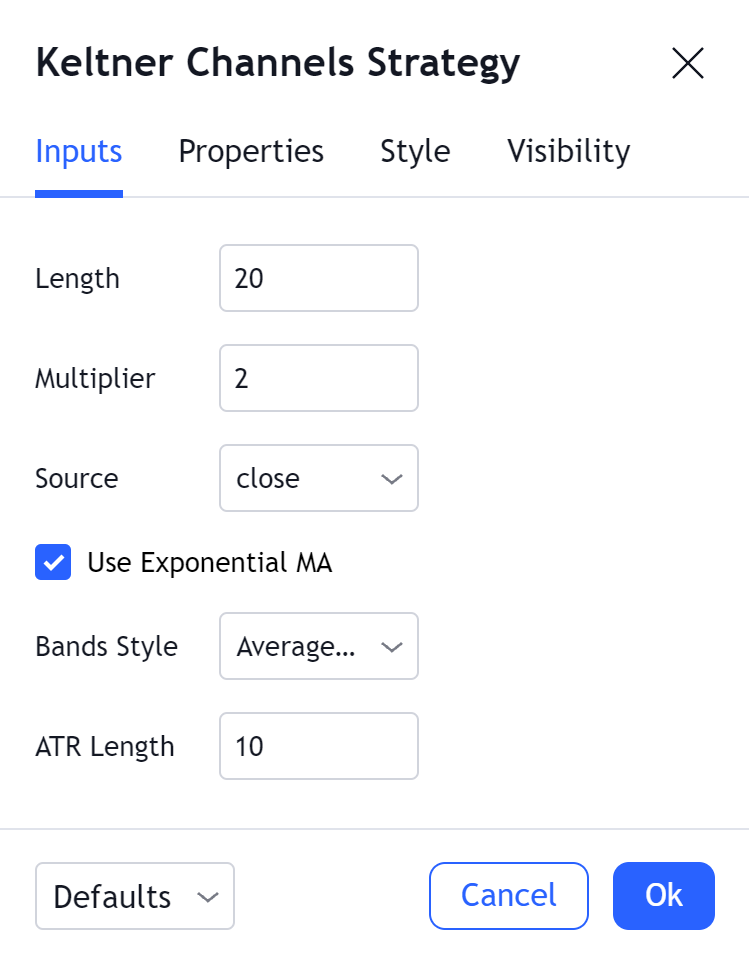# Keltner Channels Strategy

#### Definition

The Keltner Channels Strategy is based on the Keltner Channels indicator. The strategy uses the Keltner Channels as a band that dictates its investing logic: the strategy enters long when the chart crosses the upper band and switches to short once it crosses the lower band.

The underlying Keltner Channels indicator is described in detail in its respective Help Center article.

#### Inputs##### Length

The time period to be used in calculating the MA which creates the base for the Upper and Lower Envelopes (20 is the default).

##### Multiplier

The multiplier to be applied to the bands.

##### Source

Determines what data from each bar will be used in calculations. Close is the default.

##### Use Exponential MA

Determines if a simple or exponential moving average will be used for the basis.

##### Bands Style

Determines if high/low range, True Range or Average True Range will be used for the bands.

##### ATR Length

If the Bands Style is Average True Range, this setting specifies the length of the ATR calculation.